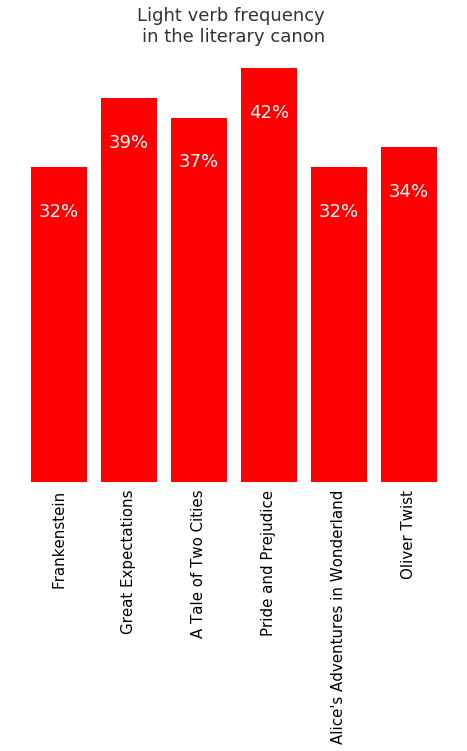Let’s take the previous analysis of a single text and formalize it so we can use it against many texts. From there we’ll explore light verb use across many texts.

We’re going to combine the find_light_verbs function from Light Verbs 1 with the class we made for Books. This will make comparisons across many texts very simple.

from itertools import compress
import matplotlib.pyplot as plt
import numpy as np
import nltk
import get_gutenberg

# Let's grab our list of all the light verbs
file = 'corpora/light_verbs.txt'
with open(file, 'r') as f:
print(light_verbs)

['be', 'am', "'m", 'is', 'are', "'re", 'wa', 'were', 'been', 'have', 'ha', 'had', "'ve", 'do', 'doe', 'did', 'done', 'go', 'goe', 'went', 'gone', 'give', 'gave', 'given', 'put', 'take', 'took', 'taken', 'feel', 'felt', 'begin', 'began', 'begun', 'get', 'got', 'make', 'put', '']


We’re going to use the class for getting text from Project Gutenberg we made previously.

books = [get_gutenberg.Book('Frankenstein', 'Mary Shelley', 'http://www.gutenberg.org/cache/epub/84/pg84.txt'),
get_gutenberg.Book('Great Expectations', 'Charles Dickens', 'http://www.gutenberg.org/files/1400/1400-0.txt'),
get_gutenberg.Book('A Tale of Two Cities', 'Charles Dickens', 'https://www.gutenberg.org/files/98/98-0.txt'),
get_gutenberg.Book('Pride and Prejudice', 'Jane Austen', 'https://www.gutenberg.org/files/1342/1342-0.txt'),
get_gutenberg.Book("Alice's Adventures in Wonderland", 'Lewis Carroll', 'https://www.gutenberg.org/files/11/11-0.txt'),
get_gutenberg.Book('Oliver Twist', 'Mark Twain', 'http://www.gutenberg.org/cache/epub/730/pg730.txt')]

all_tokens = []
for book in books:
all_tokens.append(book.tokens)

Frankenstein file already exists
Extracting text from file
Extracting text from file
A Tale of Two Cities file already exists
Extracting text from file
Pride and Prejudice file already exists
Extracting text from file
Extracting text from file
Extracting text from file


Use the function we built in the previous notebook.

def find_light_verbs(tokens):
'''
This function accepts tokens as an input and returns:
light_verbs_in_text - a list of all the light verbs in the text
light_verb_posi - a list of Booleans for each element in words of whether it is a light verb or not
num_verbs - the total number of verbs in the text
'''
# Start by taking all the text using NLTK's default tagger
tags = nltk.pos_tag(tokens)

words = []
word_mapper = []
for idx, token in enumerate(tokens):
# Use "isalnum" to check if character is alphanumberic (decimal or letter, aka not punctuation)
if token.isalnum() or (token in ["'m", "'re", "'ve", "'d", "'ll"]):
words.append(token.lower())
word_mapper.append(idx)
# Now let's create a stemmer and stem the words
stemmer = nltk.PorterStemmer()
stems = [stemmer.stem(word) for word in words]
all_verbs = [None] * len(stems)
light_verb_posi = [None] * len(stems)
verb_pos = ['VB', 'VBD', 'VBG', 'VBN', 'VBP', 'VBZ']
for idx, _ in enumerate(stems):
all_verbs[idx] = tags[word_mapper[idx]] in verb_pos
light_verb_posi[idx] = all_verbs[idx] and stems[idx] in light_verbs
num_verbs = sum(all_verbs)
light_verbs_in_text = list(compress(words, light_verb_posi))
return light_verbs_in_text, light_verb_posi, num_verbs

all_percents = []
for book in books:
list_of_light_verbs, loc, num_verbs = find_light_verbs(book.tokens)
percent_light_verbs = len(list_of_light_verbs)/num_verbs
all_percents.append(percent_light_verbs)
print("In {title}, {perc:.2%} of the {num_verbs} verbs are light.".format(title=book.title, perc=percent_light_verbs, num_verbs=num_verbs))

In Frankenstein, 32.02% of the 14159 verbs are light.
In Great Expectations, 38.76% of the 36586 verbs are light.
In A Tale of Two Cities, 37.45% of the 24877 verbs are light.
In Pride and Prejudice, 42.19% of the 24347 verbs are light.
In Alice's Adventures in Wonderland, 32.21% of the 5892 verbs are light.
In Oliver Twist, 33.81% of the 29558 verbs are light.


Now let’s plot them all so we can see how much variation there is.

plt.figure(figsize=(8, 8))

titles = [b.title for b in books]
x = np.arange(len(titles))
graph_verbs = [round(100 * percent_light) for percent_light in all_percents]

# create the bars
bars = plt.bar(x, graph_verbs, align='center', linewidth=0, color='red')

# soften all labels by turning grey
plt.xticks(x, titles, rotation='vertical', fontsize=15)
# remove the Y label since bars are directly labeled
#plt.ylabel('% Popularity', alpha=0.8)
plt.title('Light verb frequency \nin the literary canon', alpha=0.8, fontsize=18)

# remove all the ticks (both axes), and tick labels on the Y axis
plt.tick_params(top='off', bottom='off', left='off', right='off', labelleft='off', labelbottom='on')

# remove the frame of the chart
for spine in plt.gca().spines.values():
spine.set_visible(False)

# direct label each bar with Y axis values
for bar in bars:
plt.gca().text(bar.get_x() + bar.get_width()/2, bar.get_height() - 5, str(int(bar.get_height())) + '%',
ha='center', color='w', fontsize=18)
plt.show()
plt.savefig('light_verbs_usage')Judging from this, it looks like anywhere between 30-45% of a text’s verb can be light verbs. If you have more than that, perhaps try to substitute in some more action verbs to drive the writing along. If you have fewer than 30%, perhaps the writing is getting too complex.